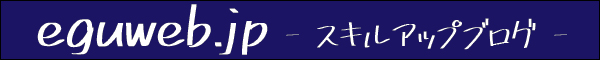# [Spreadsheet] How to dynamically get the last row of a column with data entered

This is a method that can be used when you want to know the last row where data is entered.

## A simple way is the COUNTA function

A simple way is to use the COUNTA function.

COUNTA – Google Docs Editors HelpReturns the number of values ​​in the dataset. Usage example COUNTA(A2:A100,B2:B100,4,26) COUNTA(1,2,3,4,5,C6:C20) Syntax COUNTA(value1, ) value1 – count the number

Returns the number of values ​​in the COUNTA
dataset.

COUNTA(value1, [value2, …])

Put =counta() and put the range inside.

At this time, the point is not to enter the cell number at the end of the range (enter only the alphabet of the column) .

Then the spreadsheet will automatically recognize the last row .

So if the number of rows increases and the data increases.

The value of the part containing the function also changes dynamically.

## Add the upper line (+2 in this case)

After that, if you are counting from the 3rd line, add 2.

It’s okay to count from A1, but in case the A1 cell is a blank cell, for example, the cells are shifted and added.

Now we have the last line number!

## summary

You have now dynamically retrieved the last row of a column that has data.

What if there are blank lines? There is a problem, so I would like to try it in another article about that point.